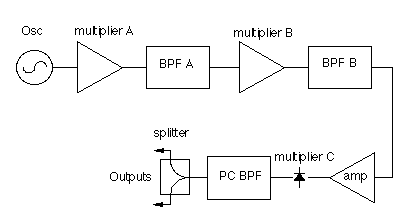Local Oscillators for 902 thru 2400 MHz

Block Diagram of Local OscillatorsFrequency-IF Oscillator multiplier A multiplier B multiplier C 902-28 109.250 X2=218.5 X2=437 X2=874 1296-28 105.666 X2=211.333 X2=422.666 X3=1268 1296-144 96.000 X2=192 X2=384 X3=1152 2304-28 94.833 X2=189.666 X3=569 X4=2276 2304-144 90.000 X2=180 X3=540 X4=2160

Local oscillators are used to up & down convert signals at virtually any frequency. The purity of these signals must be good otherwise un-wanted signals can be converted and mixed with the desired signals. The local oscillator designs presented here are very clean and when used inconjunction with a well filtered receiver front end will give unparalleled performance.

The following LOs are easy to build and offer versatility in that the individual stages can be used in other configurations and applications without problems. All stages are 50 Ohm input and output impedances. The various multipliers can be trusted for their stability and purity.

These application notes were originally presented at the 1995 MicroWave Update in Dallas, TX.

go to schematic diagram

go to components layouts

go to PC BPFs